Search by Topic

Resources tagged with Angles - points, lines and parallel lines similar to The Time Is ...:

Filter by: Content type:
Age range:
Challenge level:

There are 41 results

Broad Topics > Angles, Polygons, and Geometrical Proof > Angles - points, lines and parallel lines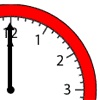Sweeping Hands

Age 7 to 11 Challenge Level:

Use your knowledge of angles to work out how many degrees the hour and minute hands of a clock travel through in different amounts of time.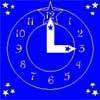Watch the Clock

Age 7 to 11 Challenge Level:

During the third hour after midnight the hands on a clock point in the same direction (so one hand is over the top of the other). At what time, to the nearest second, does this happen?Clock Hands

Age 7 to 11 Challenge Level:

This investigation explores using different shapes as the hands of the clock. What things occur as the the hands move.Take the Right Angle

Age 7 to 11 Challenge Level:

How many times in twelve hours do the hands of a clock form a right angle? Use the interactivity to check your answers.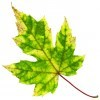Being Resourceful - Primary Geometry

Age 5 to 11 Challenge Level:

Geometry problems at primary level that require careful consideration.Being Collaborative - Primary Geometry

Age 5 to 11 Challenge Level:

Geometry problems for primary learners to work on with others.Being Curious - Primary Geometry

Age 5 to 11 Challenge Level:

Geometry problems for inquiring primary learners.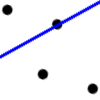Dotty Relationship

Age 7 to 11 Challenge Level:

Can you draw perpendicular lines without using a protractor? Investigate how this is possible.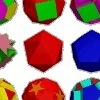Cylinder Cutting

Age 7 to 11 Challenge Level:

An activity for high-attaining learners which involves making a new cylinder from a cardboard tube.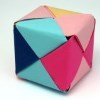Being Resilient - Primary Geometry

Age 5 to 11 Challenge Level:

Geometry problems at primary level that may require resilience.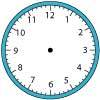Right Time

Age 11 to 14 Challenge Level:

At the time of writing the hour and minute hands of my clock are at right angles. How long will it be before they are at right angles again?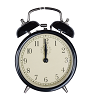On Time

Age 11 to 14 Challenge Level:

On a clock the three hands - the second, minute and hour hands - are on the same axis. How often in a 24 hour day will the second hand be parallel to either of the two other hands?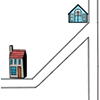Six Places to Visit

Age 7 to 11 Challenge Level:

Can you describe the journey to each of the six places on these maps? How would you turn at each junction?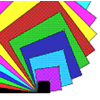Overlapping Squares

Age 7 to 11 Challenge Level:

Have a good look at these images. Can you describe what is happening? There are plenty more images like this on NRICH's Exploring Squares CD.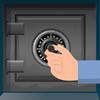How Safe Are You?

Age 7 to 11 Challenge Level:

How much do you have to turn these dials by in order to unlock the safes?LOGO Challenge 7 - More Stars and Squares

Age 11 to 16 Challenge Level:

Can you use LOGO to create a systematic reproduction of a basic design? An introduction to variables in a familiar setting.Right Angles

Age 11 to 14 Challenge Level:

Can you make a right-angled triangle on this peg-board by joining up three points round the edge?Polygon Rings

Age 11 to 14 Challenge Level:

Join pentagons together edge to edge. Will they form a ring?Triangles in Circles

Age 11 to 14 Challenge Level:

Can you find triangles on a 9-point circle? Can you work out their angles?Dotty Circle

Age 7 to 11 Challenge Level:

Watch this film carefully. Can you find a general rule for explaining when the dot will be this same distance from the horizontal axis?Subtended Angles

Age 11 to 14 Challenge Level:

What is the relationship between the angle at the centre and the angles at the circumference, for angles which stand on the same arc? Can you prove it?Estimating Angles

Age 7 to 14 Challenge Level:

How good are you at estimating angles?Tessellating Hexagons

Age 11 to 14 Challenge Level:

Which hexagons tessellate?Olympic Turns

Age 7 to 11 Challenge Level:

This task looks at the different turns involved in different Olympic sports as a way of exploring the mathematics of turns and angles.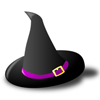Witch's Hat

Age 11 to 16 Challenge Level:

What shapes should Elly cut out to make a witch's hat? How can she make a taller hat?Making Maths: Equilateral Triangle Folding

Age 7 to 14 Challenge Level:

Make an equilateral triangle by folding paper and use it to make patterns of your own.Which Solids Can We Make?

Age 11 to 14 Challenge Level:

Interior angles can help us to work out which polygons will tessellate. Can we use similar ideas to predict which polygons combine to create semi-regular solids?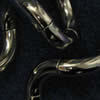Age 7 to 16 Challenge Level:

A metal puzzle which led to some mathematical questions.Robotic Rotations

Age 11 to 16 Challenge Level:

How did the the rotation robot make these patterns?Interacting with the Geometry of the Circle

Age 5 to 16

Jennifer Piggott and Charlie Gilderdale describe a free interactive circular geoboard environment that can lead learners to pose mathematical questions.Semi-regular Tessellations

Age 11 to 16 Challenge Level:

Semi-regular tessellations combine two or more different regular polygons to fill the plane. Can you find all the semi-regular tessellations?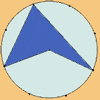Angle Measurement: an Opportunity for Equity

Age 11 to 16

Suggestions for worthwhile mathematical activity on the subject of angle measurement for all pupils.Same Length

Age 11 to 16 Challenge Level:

Construct two equilateral triangles on a straight line. There are two lengths that look the same - can you prove it?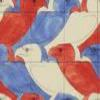Maurits Cornelius Escher

Age 7 to 14

Have you ever noticed how mathematical ideas are often used in patterns that we see all around us? This article describes the life of Escher who was a passionate believer that maths and art can be. . . .Pythagoras

Age 7 to 14

Pythagoras of Samos was a Greek philosopher who lived from about 580 BC to about 500 BC. Find out about the important developments he made in mathematics, astronomy, and the theory of music.LOGO Challenge 1 - Star Square

Age 7 to 16 Challenge Level:

Can you use LOGO to create this star pattern made from squares. Only basic LOGO knowledge needed.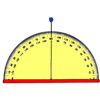Making Maths: Clinometer

Age 11 to 14 Challenge Level:

Make a clinometer and use it to help you estimate the heights of tall objects.LOGO Challenge 8 - Rhombi

Age 7 to 16 Challenge Level:

Explore patterns based on a rhombus. How can you enlarge the pattern - or explode it?Round and Round and Round

Age 11 to 14 Challenge Level:

Where will the point stop after it has turned through 30 000 degrees? I took out my calculator and typed 30 000 ÷ 360. How did this help?Shogi Shapes

Age 11 to 14 Challenge Level:

Shogi tiles can form interesting shapes and patterns... I wonder whether they fit together to make a ring?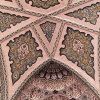Angles Inside

Age 11 to 14 Challenge Level:

Draw some angles inside a rectangle. What do you notice? Can you prove it?